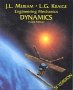# Physics - Kinematics - Linear Acceleration

If an object is moving at a constant acceleration (as it would be if acted on by a constant force see forces )

Its acceleration is given by:

a = dv / dT

or integrating:

v = ∫a dT

In the more general case of translation in 3 dimensions:

[a] =#### Constant Acceleration (constant force)

If acceleration is constant, which happens when force is constant, so it is a common occurrence, for example when a body is falling in a gravity field.

These equations for uniform acceleration can be solved analytically to give the following equations which relate p, p0, v, v0, t and a in different ways:

• v = v0 + a*t
• p = p0 + v0 t + ½ a t2
• v2 = v02 + 2 * a * p
 where: other definitions symbol description type units v0 initial velocity vector m/s v final velocity vector m/s a acceleration constant vector m/s2 t time scalar s p final position vector m p 0 position at t=0 vector m

These equations can be derived using calculus as follows:

Velocity is the rate if change of position:

a = dv/dt

So integrating both sides gives:

v = ∫a dt

so if a is constant:

v = v0 + a*t

I seem to remember that when I was at school this was written as:

v = u + a t

So integrating acceleration once gives the velocity, to get the position we need to integrate again:

p = ∫v dt

p = ∫(v0 + a*t) dt

p = p0 + v0 t + ½ a t2

#### Variable Acceleration - approximate methods

If we have an equation for the acceleration, as a function of time, we can apply integration to find the velocity and position, if we don't then we can use approximate methods such as finite difference method, Eulers Method or Runge-Kutta Method. If we are animating a computer simulation then this can be a very good method because we need to generate the position for each frame anyway, so is is much easier to generate the next frame from the frame before it.

vn+1 = vn + a * dt

 where: other definitions symbol description type units vn+1 velocity at frame n+1 vector m/s vn velocity at frame n vector m/s a acceleration vector m/s2 dt time between frame n and frame n+1 scalar s

and summing again:

pn+1 = pn + vn * dt

 where: other definitions symbol description type units pn+1 position at frame n+1 vector m pn position at frame n vector m vn velocity at frame n vector m/s dt time between frame n and frame n+1 scalar s

These approximations can be made more accurate by using Eulers Method or Runge-Kutta Method

## Representing Acceleration in program

Acceleration in 3D space can be held in a 3D vector (see class sfvec3f). For an example of how this might be used in a scenegraph node, see here.

 metadata block see also: Correspondence about this page Book Shop - Further reading. Where I can, I have put links to Amazon for books that are relevant to the subject, click on the appropriate country flag to get more details of the book or to buy it from them.Engineering Mechanics Vol 2: Dynamics - Gives theory for rigid dynamics, aims to allow prediction of effects of force and motion. Includes rotating frame of reference. Lots of colour diagrams, I guess its college / University level. Commercial Software Shop Where I can, I have put links to Amazon for commercial software, not directly related to the software project, but related to the subject being discussed, click on the appropriate country flag to get more details of the software or to buy it from them.

This site may have errors. Don't use for critical systems.